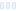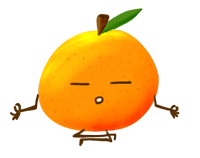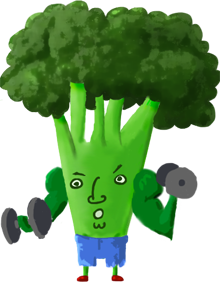# Watermelon Hydration Multiplier

## Liquid I.V.

Main info:

Watermelon Hydration Multiplier
Liquid I.V.
1 stick
45 Calories
12 g
0 g
0 g

0 g
0 mg
0 g
510 mg
11 g
0 g

Percent calories from...
Nutrition Facts
For a Serving Size of (g)
How many calories are in Watermelon Hydration Multiplier? Amount of calories in Watermelon Hydration Multiplier: Calories Calories from Fat (%)
% Daily Value *
How much fat is in Watermelon Hydration Multiplier? Amount of fat in Watermelon Hydration Multiplier: Total Fat
How much sodium is in Watermelon Hydration Multiplier? Amount of sodium in Watermelon Hydration Multiplier: Sodium
How much potassium is in Watermelon Hydration Multiplier? Amount of potassium in Watermelon Hydration Multiplier: Potassium
How many carbs are in Watermelon Hydration Multiplier? Amount of carbs in Watermelon Hydration Multiplier: Carbohydrates
How many net carbs are in Watermelon Hydration Multiplier? Amount of net carbs in Watermelon Hydration Multiplier: Net carbs
How much sugar is in Watermelon Hydration Multiplier? Amount of sugar in Watermelon Hydration Multiplier: Sugar
How much fiber is in Watermelon Hydration Multiplier? Amount of fiber in Watermelon Hydration Multiplier: Fiber
How much protein is in Watermelon Hydration Multiplier? Amount of protein in Watermelon Hydration Multiplier: Protein
Vitamins and minerals
How much Vitamin D is in Watermelon Hydration Multiplier? Amount of Vitamin D in Watermelon Hydration Multiplier: Vitamin D
How much Calcium is in Watermelon Hydration Multiplier? Amount of Calcium in Watermelon Hydration Multiplier: Calcium
How much Iron is in Watermelon Hydration Multiplier? Amount of Iron in Watermelon Hydration Multiplier: Iron
Fatty acids
Amino acids
* The Percent Daily Values are based on a 2,000 calorie diet, so your values may change depending on your calorie needs.Loading similar foods...
Note: Any items purchased after clicking our Amazon buttons will give us a little referral bonus. If you do click them, thank you!Be cool

- Zen orangeI never skip arm day

- Buff broccoli